# Midpoint 6

FM=8a+1, FG=42, a=? Point M is the midpoint of FG. Find unknown a.

Result

a =  2.5

#### Solution:

8*a+1 = 42/2

16a = 40

a = 52 = 2.5

Calculated by our simple equation calculator.

Leave us a comment of example and its solution (i.e. if it is still somewhat unclear...):

Showing 0 comments:Be the first to comment!#### To solve this example are needed these knowledge from mathematics:

Looking for help with calculating arithmetic mean? Looking for a statistical calculator? Do you have a linear equation or system of equations and looking for its solution? Or do you have quadratic equation? Do you want to convert length units?

## Next similar examples:

1. Center of gravityThe mass points are distributed in space as follows - specify by coordinates and weight. Find the center of gravity of the mass points system: A1 [14; -2; 5] m1 = 10.2 kg A2 [-2; -16; 7] m2 = 13.6 kg A3
2. Centroid - two bodiesA body is composed of a 0.8 m long bar and a sphere with a radius of 0.1m attached so that its center lies on the longitudinal axis of the bar. Both bodies are of the same uniform material. The sphere is twice as heavy as the bar. Find the center of gravit
3. Contestants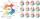In the competition it was possible to get 0-5 points. In fact, 5 contestants of the 15 best contestants has earned 5 points or 4 points (which have won 10 contestants). The number of contestants who earned 3 points was the same as the number of contestan
4. Athletic competitionIn a 400 meter athletic competition, a participant covers the distance as given below. find the average speed? first 80 meters 10 m/s next 240 meters 7.5 m/s last 80 meters 10 m/s
5. US GDP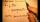Consider the following dataset , which contsins the domestic US gross in millions of the top grossing movie over the last 5 years. 300,452,513,550,780 I. Find the Mean of the Dataset II. Find the Squared deviation of the second observation from the mean I
6. Standard deviationFind standard deviation for dataset (grouped data): Age (years) No. Of Persons 0-10 15 10-20 15 20-30 23 30-40 22 40-50 25 50-60 10 60-70 5 70-80 10
7. Ten cashiers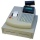Ten cashiers are open at Tesco. Customers wait an average of 15 minutes. How many other cashiers have to open to reduce the waiting time by 4 minutes?
8. The poolThe pool has a volume of 40 m3 and the water temperature is 20 °C. How much water at 100 °C should we pour into the pool to increase the water temperature by 5 °C?
9. Certificate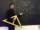There is 31 students in a class. From mathematics was'nt worse mark than 2. Average mark in mathematics was 2. How many students have mark 1 and how many mark 2?
10. Uphill and downhillThe cyclist moves uphill at a constant speed of v1 = 10 km/h. When he reaches the top of the hill, he turns and passes the same track downhill at a speed of v2 = 40 km/h. What is the average speed of a cyclist?
11. WaterMix 21 l of water with temperature of 38 °C, 67 l warm of 34 °C and 14 l water of 32 °C. What is the temperature of the mixed water immediately after mixing?
12. Average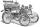What is the average speed of the car, where half of the distance covered passed at speed 66 km/h and the other half at 86 km/h.
13. Annual incomeThe annual incomes (in thousands of \$) of fifteen families is: 60, 80, 90, 96, 120, 150, 200, 360, 480, 520, 1060, 1200, 1450, 2500, 7200 Calculate harmonic and geometric mean.
14. CarpentersCarpenters 1 and 2 spend 10 days and 5 days respectively to make one table. If 50 tables were made by the first carpenter and 30 tables were made by the second carpenter, What is the average time spent on the products?
15. SD - meanMean is 10 and standard deviation is 3.5. If the data set contains 40 data values, approximately how many of the data values will fall within the range 6.5 to 13.5?
16. Controller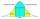Output Controller of the company in the control of 50 randomly selected products found that 37 of them had no defect 8 has only one flaw, three had two defects, and two products had three defects. Determine the standard deviation and coefficient of variati
17. Normal DistributionAt one college, GPA's are normally distributed with a mean of 3.1 and a standard deviation of 0.4. What percentage of students at the college have a GPA between 2.7 and 3.5?